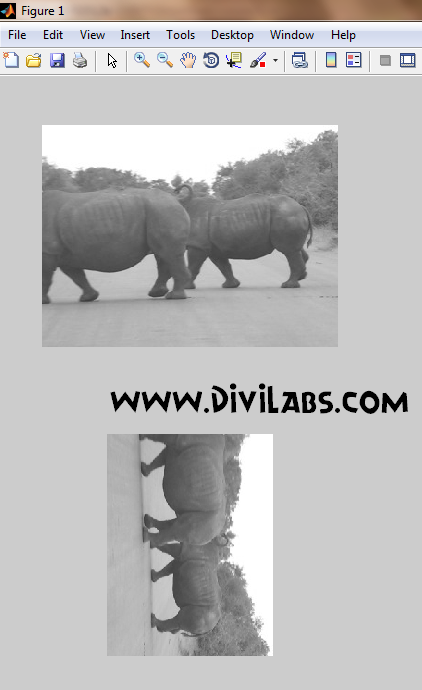# MATLAB Code To Rotate the Video Clockwise 90 degree During its Preview: MATLAB Video Processing

Its a code that we have developed in our leisure time. So we just struck with an idea to rotate the video clockwise 90 degree, & that too using MATLAB. Spent few minutes, & yes its working now!

The following MATLAB Code is To Rotate the Video Clockwise 90 degree During its Preview. You can also save this rotated video using VideoWriter class, if you want it for further use! This code will display result in GrayScale form.

MATLAB Code:

clc;
close all;
clear all;
[x, y, z, w]=size(obj1);
% to get the parameters that will be helpful to decide how the looping should be done
%x= height of video frame
%y= width of video frame
%z= color plane present in the video frame
%w= number of frames present in the video

for i=1:w
frb = rgb2gray(fr); %converting RGB frame to Grayscale Frame
frt = frb'; %performing the transpose of the frame matrix
for j=1:x/2
for k=1:y
l= frt(k,j);
frt(k,j)= frt(k,x-j+1);
frt(k,x-j+1)= l;
end
end
figure(1)
subplot(2,1,1),imshow(frb)
subplot(2,1,2),imshow(uint8(frt))
end

Screenshot:The video above, is the original one & the below one is rotated one : Video Rotation Using MATLAB